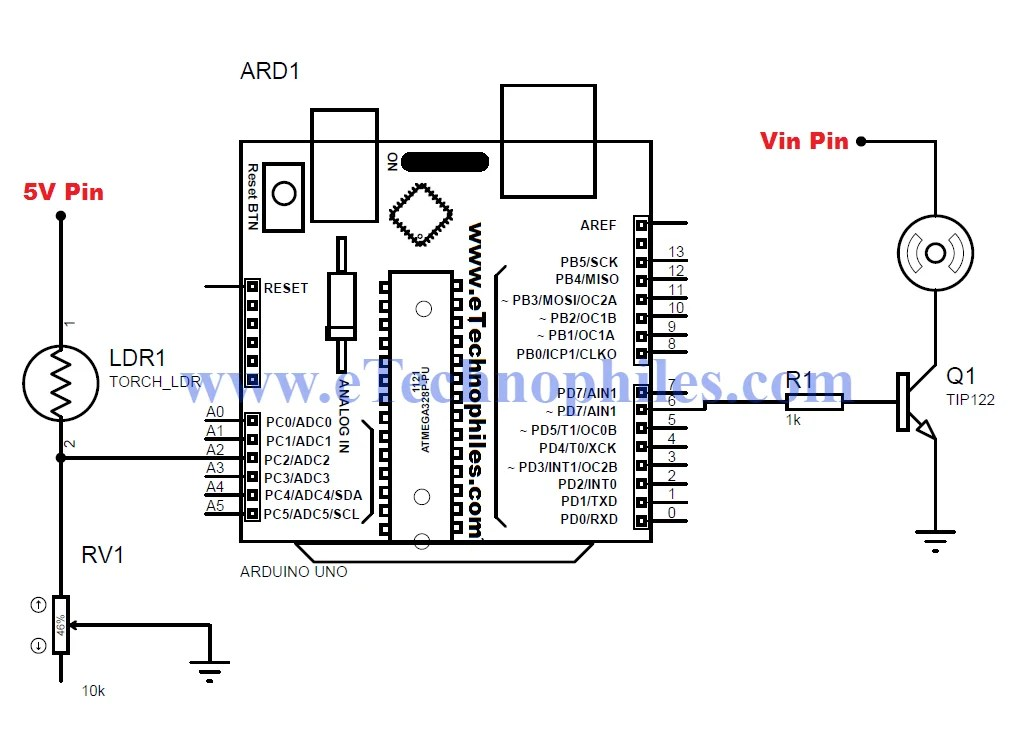# Arduino Project | Automated speed control of Motor using LDR with ArduinoIn this Arduino project, we are going to build an automatic Speed Controller of DC Motor using LDR with Arduino. This LDR based Arduino project slows down the speed of Motor as the intensity of light falling on LDR decreases and vice versa. “Arduino Project| Motor speed control using LDR with Arduino”

Note : To know more about LDR, visit this page

Components needed for this Automatic Street Light:

1. LDR

2. Arduino UNO

3. TIP122 Transistor

4. DC Motor

5. 1 kohm resistor

6. 10 k ohm potentiometer

5. Connecting wires

Terminals of Transistor TIP122:CIRCUIT DIAGRAM:Circuit of Automated Speed Motor Control using LDR based Arduino project

Arduino Code for LDR based motor speed control Arduino Project :

 int x; // input of LDR int y; // o/p Pwm value to be given to the base of the transistor to get reduced o/p voltagevoid setup() { pinMode(A0,INPUT); pinMode(6,OUTPUT); Serial.begin(9600); } void loop() { x= analogRead(A0);// analog input from the pin A0 connected to LDR and POT. end y= map(x,0,1023,0,255); // Mapping or converting the value bw minimum to maximum analog value to the pwm value analogWrite(6,y); // Writing this analog value to pin 6 which is connected to the base of the transistor to get reduced o/p voltage Serial.print(“LDR input “); Serial.print(x); Serial.print(“\t”); Serial.print(” O/P PWM value “); Serial.print(y); Serial.println(); delay(1000); }

**Explanation of the code is in comments itself.

Working of LDR based motor speed control Arduino Project:

1. When light falls on LDR, the resistance of LDR changes. This change in resistance causes a change in voltage drop across LDR. Now since potentiometer is connected in series with LDR i.e, to Analog pin A0 to Ground, the voltage drop across it also changes. This change in voltage drop is received by Analog pin A0 in the form of analog value anywhere between 0 and 1023.

2. This received analog value is then converted to PWM value between 0 and 255 by Arduino  using this piece of code:

y= map(x,0,1023,0,255); // Mapping or converting the value bw minimum to maximum analog value to the corresponding pwm value

3. This PWM value is now given to the digital pin 6 initialized as an output pin and connected to the base of the transistor.

4. The code used to write this PWM value to pin 6 is:

analogWrite(6,y); // Writing this analog value to pin 6 which is connected to the base of the transistor to get reduced o/p voltage

5. This causes the switching of the transistor according to the provided PWM value at its base.

6. And this change in switching frequency causes a change in the speed of Motor.

Hence this way Automated Motor speed Control is obtained using LDR based Arduino Project

### One Reply to “Arduino Project | Automated speed control of Motor using LDR with Arduino”

1.gooda says:

if I want to add 3 LEDs, where should the led be put